# Central Limit Theorem n Complete Tasks 1 and

• Slides: 41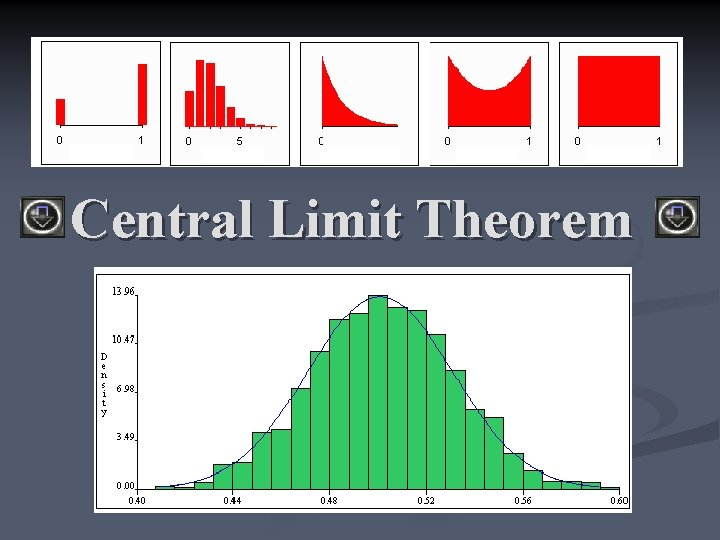Central Limit Theoremn Complete Tasks 1 and 2 on worksheet.Your task today… n Is to be a noticer n As you see each slide ask yourself: n What do I notice?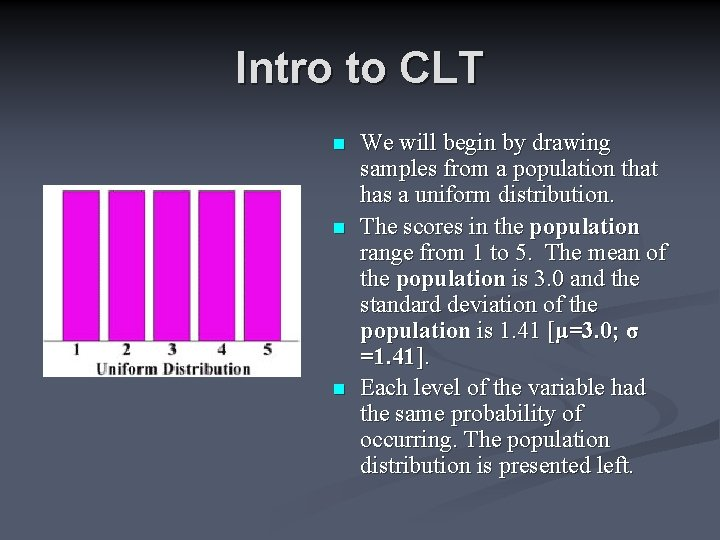Intro to CLT n n n We will begin by drawing samples from a population that has a uniform distribution. The scores in the population range from 1 to 5. The mean of the population is 3. 0 and the standard deviation of the population is 1. 41 [μ=3. 0; σ =1. 41]. Each level of the variable had the same probability of occurring. The population distribution is presented left.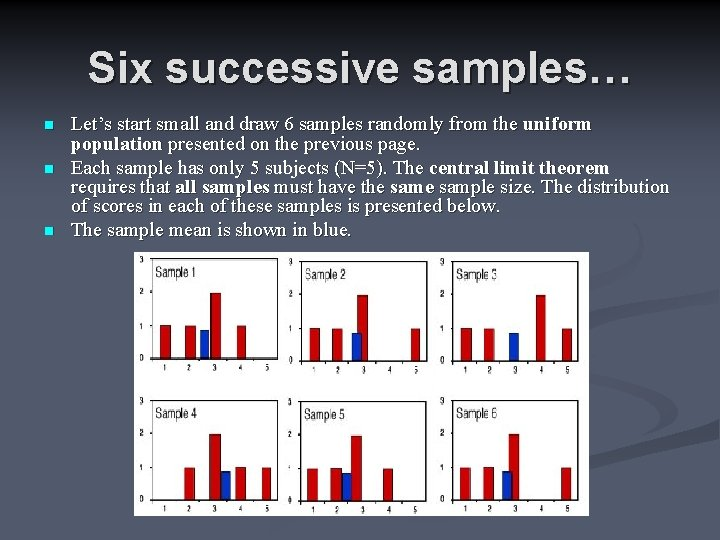Six successive samples… n n n Let’s start small and draw 6 samples randomly from the uniform population presented on the previous page. Each sample has only 5 subjects (N=5). The central limit theorem requires that all samples must have the sample size. The distribution of scores in each of these samples is presented below. The sample mean is shown in blue.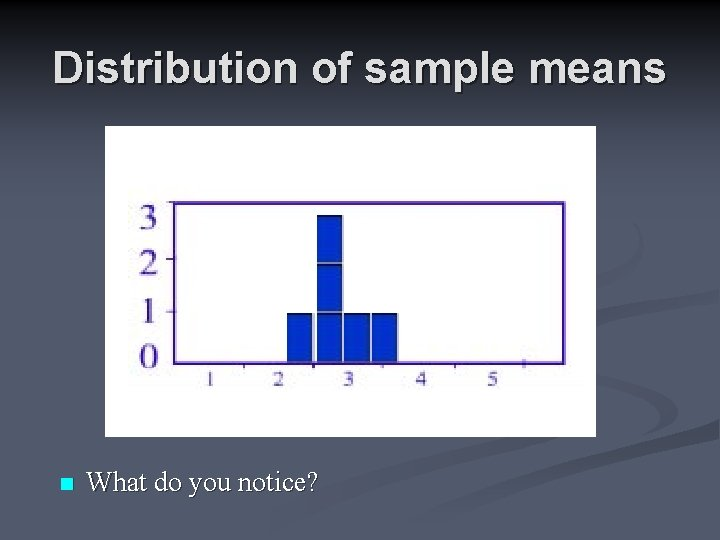Distribution of sample means n What do you notice?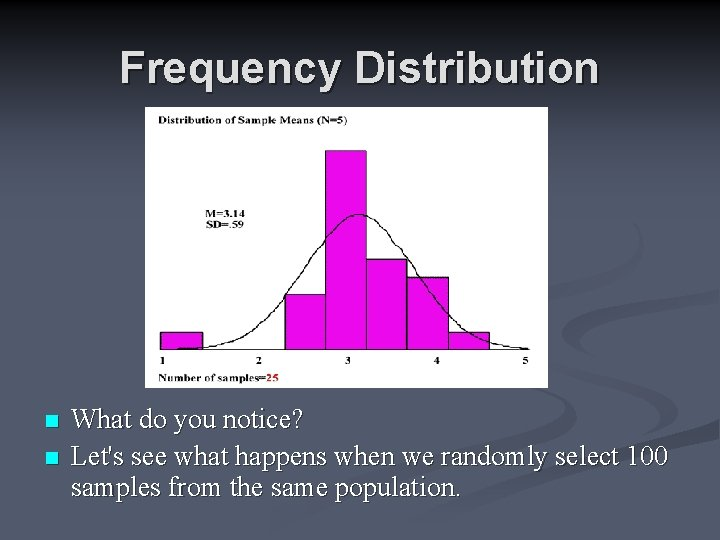Frequency Distribution n n What do you notice? Let's see what happens when we randomly select 100 samples from the same population.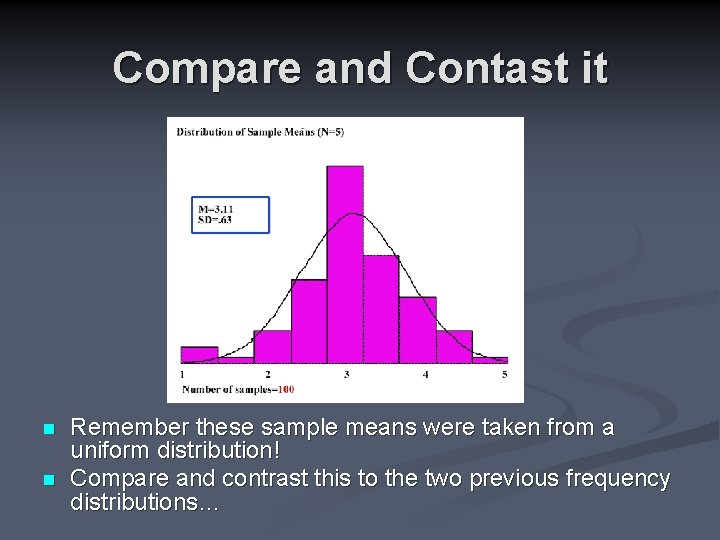Compare and Contast it n n Remember these sample means were taken from a uniform distribution! Compare and contrast this to the two previous frequency distributions…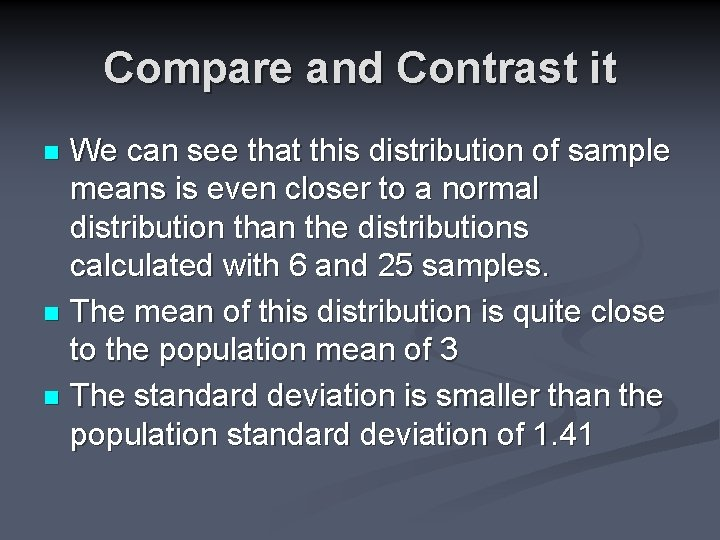Compare and Contrast it We can see that this distribution of sample means is even closer to a normal distribution than the distributions calculated with 6 and 25 samples. n The mean of this distribution is quite close to the population mean of 3 n The standard deviation is smaller than the population standard deviation of 1. 41 n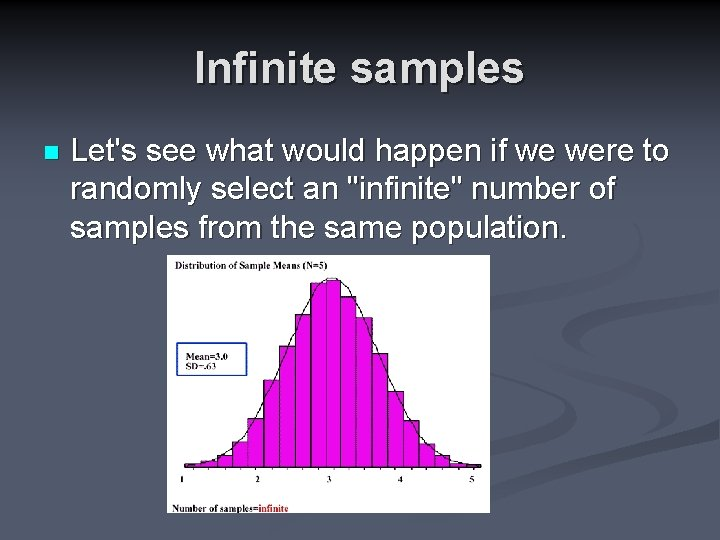Infinite samples n Let's see what would happen if we were to randomly select an "infinite" number of samples from the same population.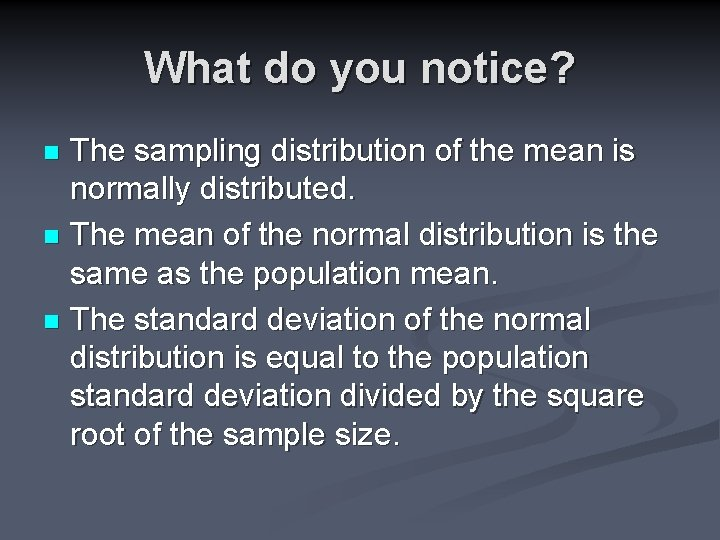What do you notice? The sampling distribution of the mean is normally distributed. n The mean of the normal distribution is the same as the population mean. n The standard deviation of the normal distribution is equal to the population standard deviation divided by the square root of the sample size. n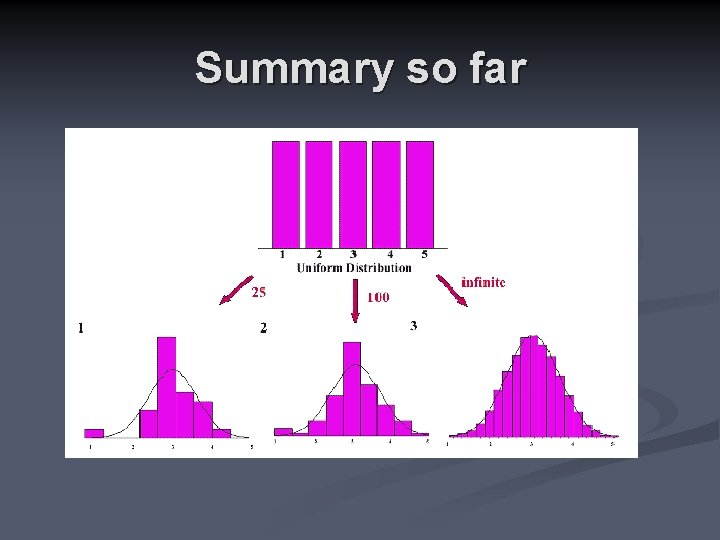Summary so farLet’s increase the sample size. n Let’s increase the sample size from 5 to 25…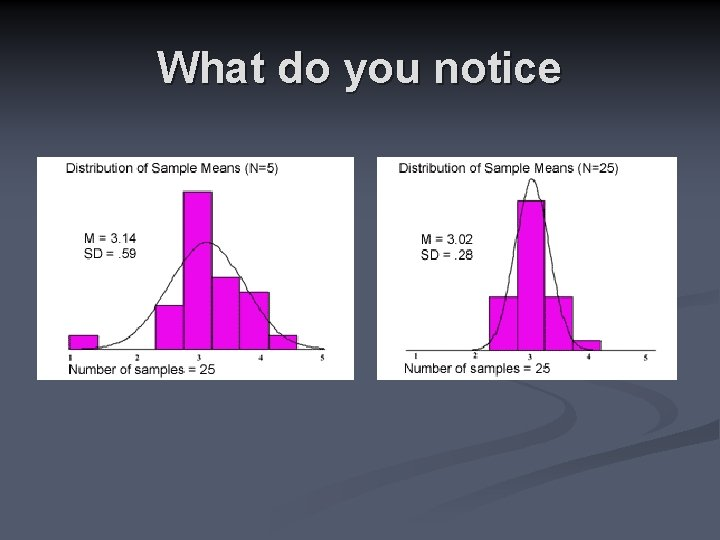What do you notice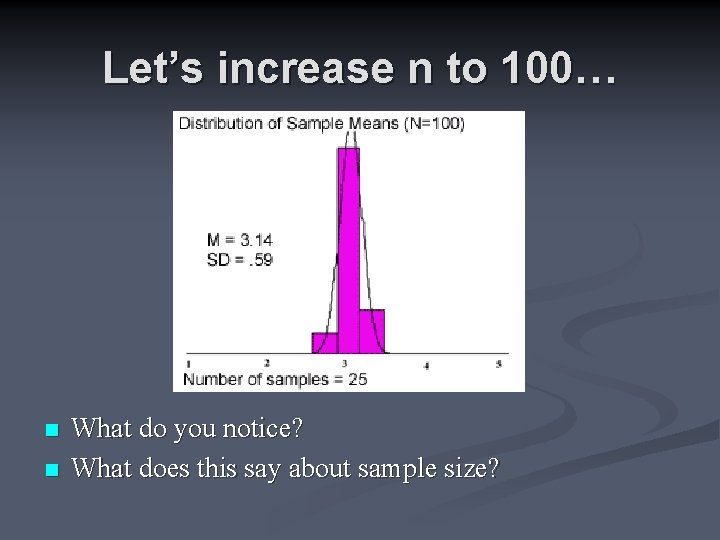Let’s increase n to 100… n n What do you notice? What does this say about sample size?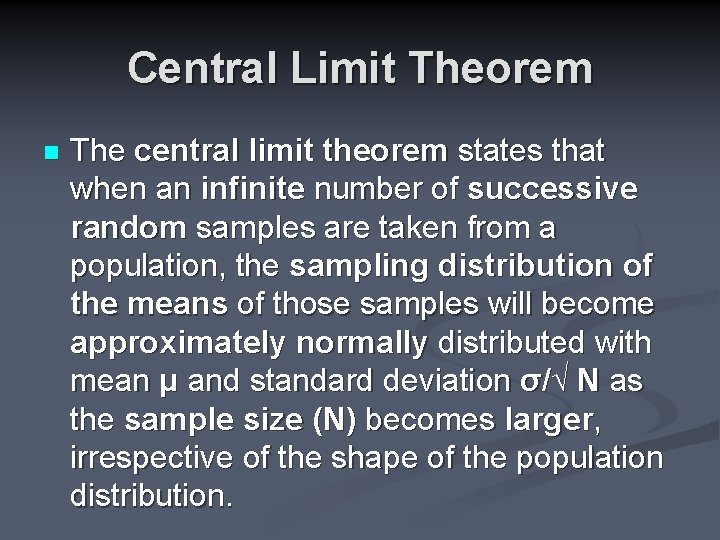Central Limit Theorem n The central limit theorem states that when an infinite number of successive random samples are taken from a population, the sampling distribution of the means of those samples will become approximately normally distributed with mean μ and standard deviation σ/√ N as the sample size (N) becomes larger, irrespective of the shape of the population distribution.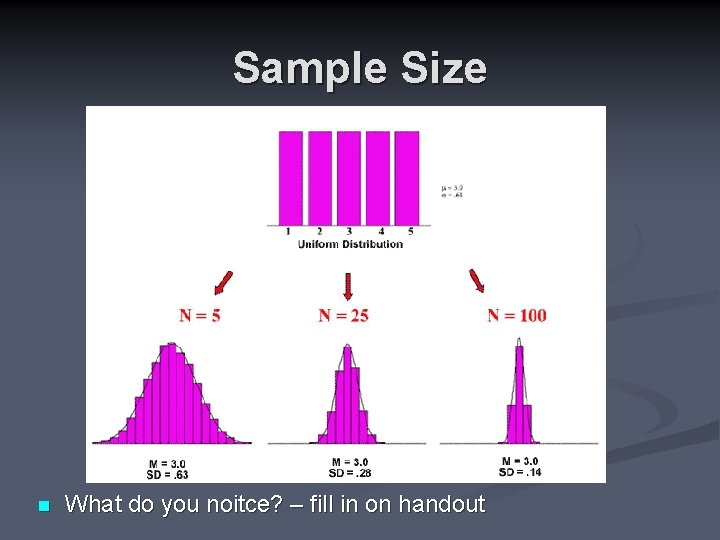Sample Size n What do you noitce? – fill in on handout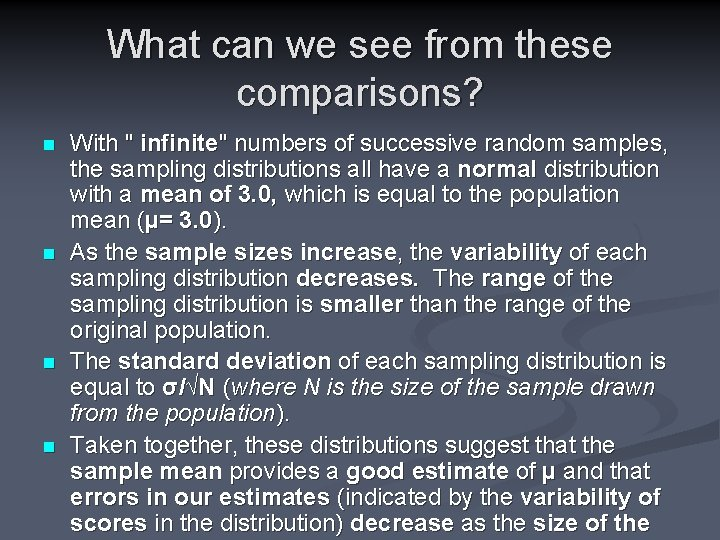What can we see from these comparisons? n n With " infinite" numbers of successive random samples, the sampling distributions all have a normal distribution with a mean of 3. 0, which is equal to the population mean (µ= 3. 0). As the sample sizes increase, the variability of each sampling distribution decreases. The range of the sampling distribution is smaller than the range of the original population. The standard deviation of each sampling distribution is equal to σ/√N (where N is the size of the sample drawn from the population). Taken together, these distributions suggest that the sample mean provides a good estimate of µ and that errors in our estimates (indicated by the variability of scores in the distribution) decrease as the size of the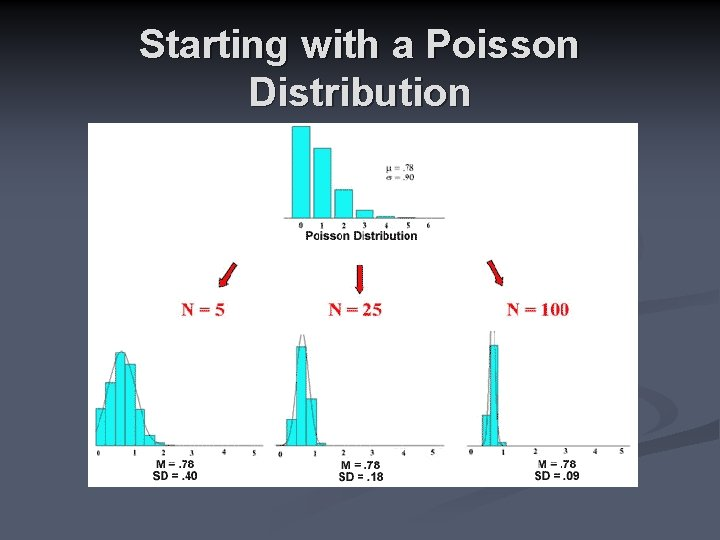Starting with a Poisson DistributionStarting with a Normal DistributionQuiz n Complete the quiz on your worksheet with a partner.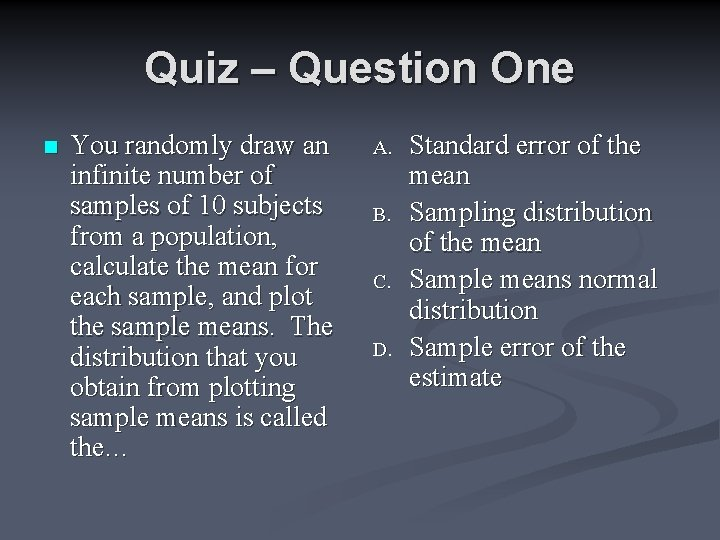Quiz – Question One n You randomly draw an infinite number of samples of 10 subjects from a population, calculate the mean for each sample, and plot the sample means. The distribution that you obtain from plotting sample means is called the… A. B. C. D. Standard error of the mean Sampling distribution of the mean Sample means normal distribution Sample error of the estimateAnswer: B. Sampling distribution of the mean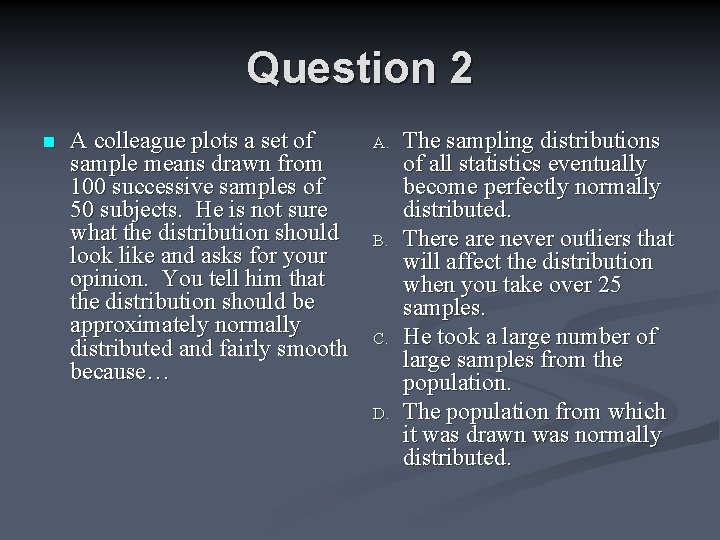Question 2 n A colleague plots a set of sample means drawn from 100 successive samples of 50 subjects. He is not sure what the distribution should look like and asks for your opinion. You tell him that the distribution should be approximately normally distributed and fairly smooth because… A. B. C. D. The sampling distributions of all statistics eventually become perfectly normally distributed. There are never outliers that will affect the distribution when you take over 25 samples. He took a large number of large samples from the population. The population from which it was drawn was normally distributed.Answer C. He took a large number of large samples from the population.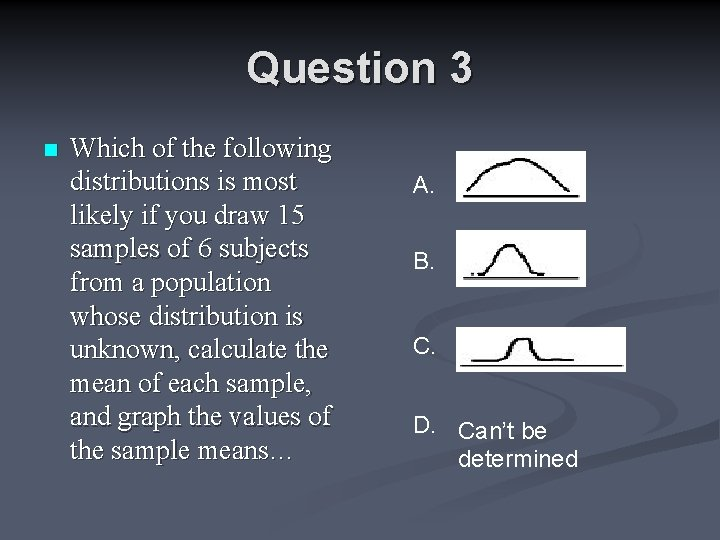Question 3 n Which of the following distributions is most likely if you draw 15 samples of 6 subjects from a population whose distribution is unknown, calculate the mean of each sample, and graph the values of the sample means… A. B. C. D. Can’t be determined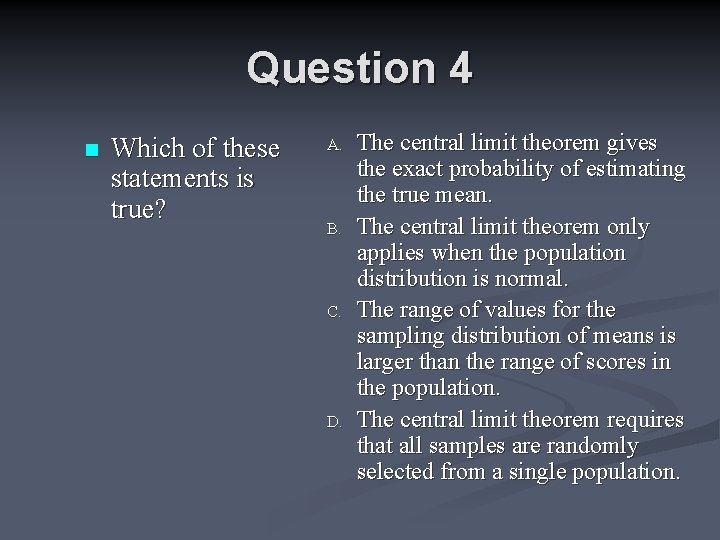Question 4 n Which of these statements is true? A. B. C. D. The central limit theorem gives the exact probability of estimating the true mean. The central limit theorem only applies when the population distribution is normal. The range of values for the sampling distribution of means is larger than the range of scores in the population. The central limit theorem requires that all samples are randomly selected from a single population.Answer: D. The central limit theorem requires that all samples are randomly selected from a single population.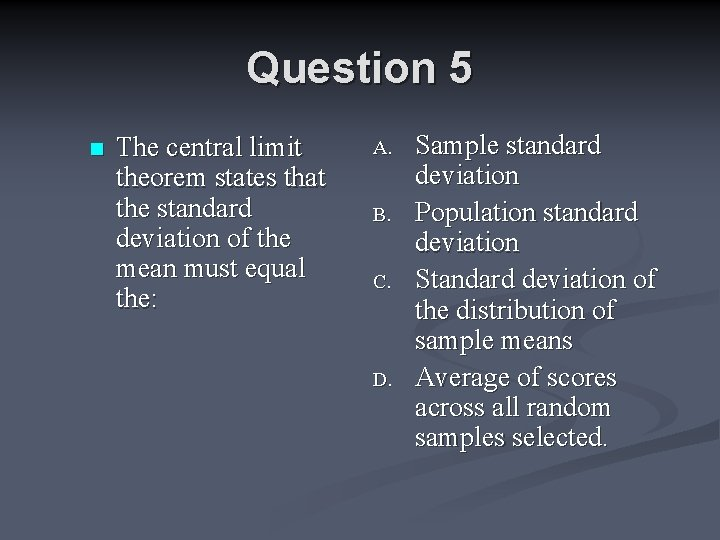Question 5 n The central limit theorem states that the standard deviation of the mean must equal the: A. B. C. D. Sample standard deviation Population standard deviation Standard deviation of the distribution of sample means Average of scores across all random samples selected.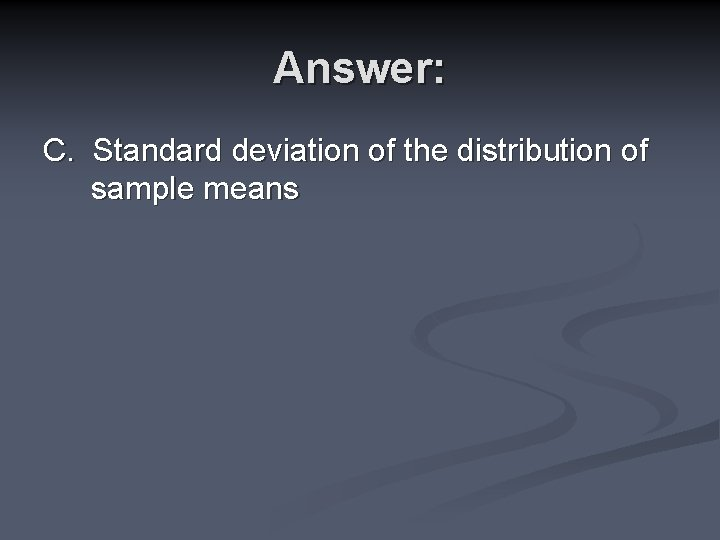Answer: C. Standard deviation of the distribution of sample meansQuestion 6 n How does the central limit theorem show us that the mean is a good estimator of the population mean (μ)? A. B. C. D. It is the easiest measure of central tendency to calculate on all the separate samples. The mean of the sampling distribution of the mean is always equal to μ. The sampling distribution of μ is always close to the sample means. Infinite sampling from a defined population is always more accurate than random sampling.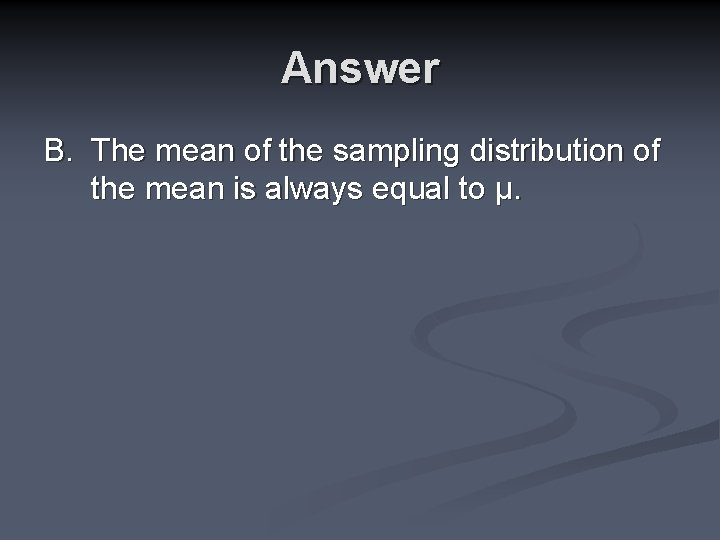Answer B. The mean of the sampling distribution of the mean is always equal to μ.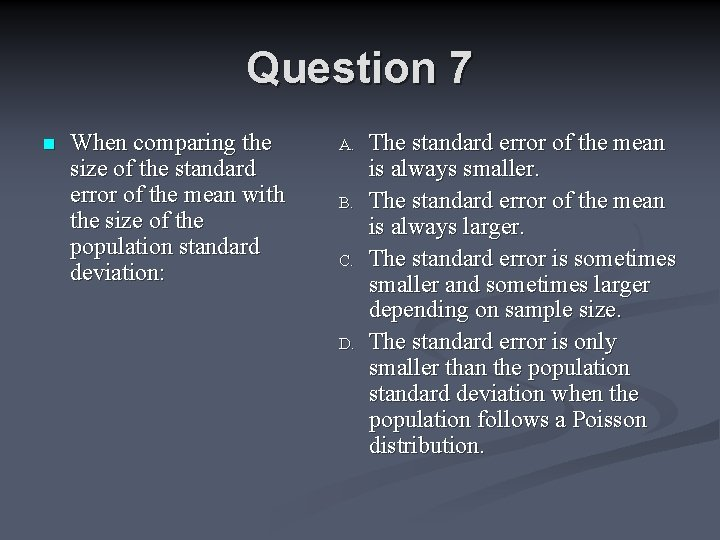Question 7 n When comparing the size of the standard error of the mean with the size of the population standard deviation: A. B. C. D. The standard error of the mean is always smaller. The standard error of the mean is always larger. The standard error is sometimes smaller and sometimes larger depending on sample size. The standard error is only smaller than the population standard deviation when the population follows a Poisson distribution.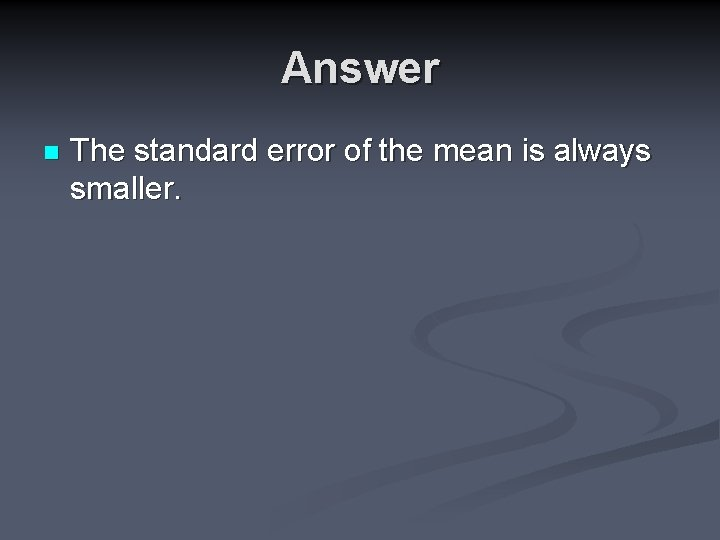Answer n The standard error of the mean is always smaller.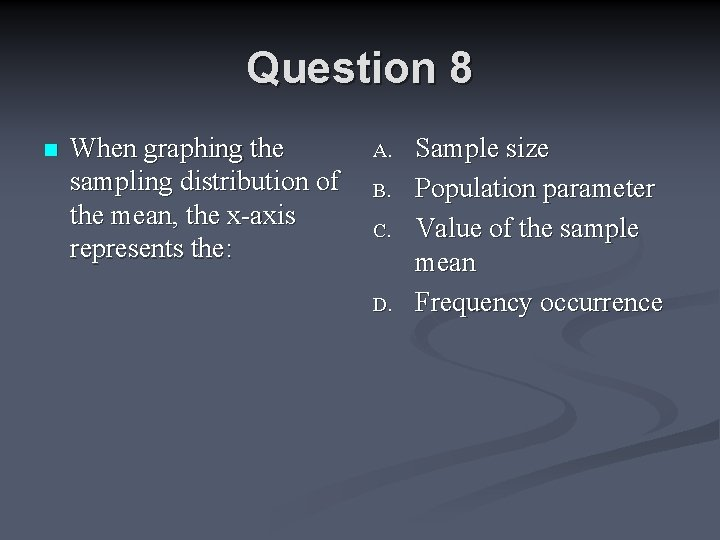Question 8 n When graphing the sampling distribution of the mean, the x-axis represents the: A. B. C. D. Sample size Population parameter Value of the sample mean Frequency occurrence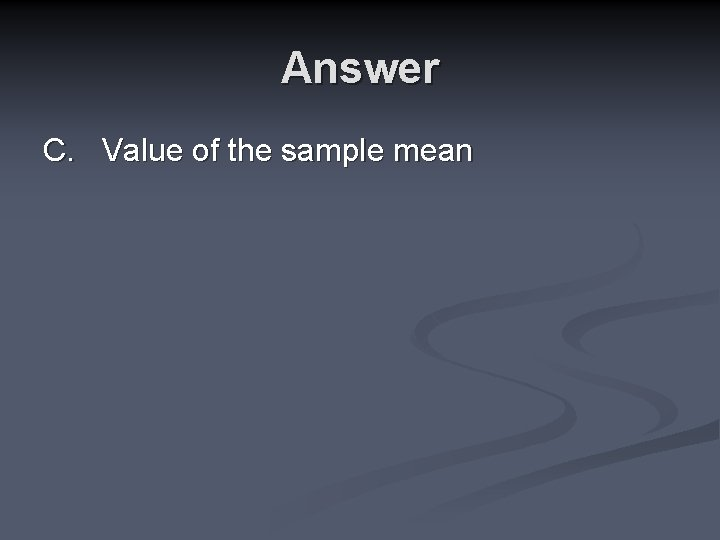Answer C. Value of the sample mean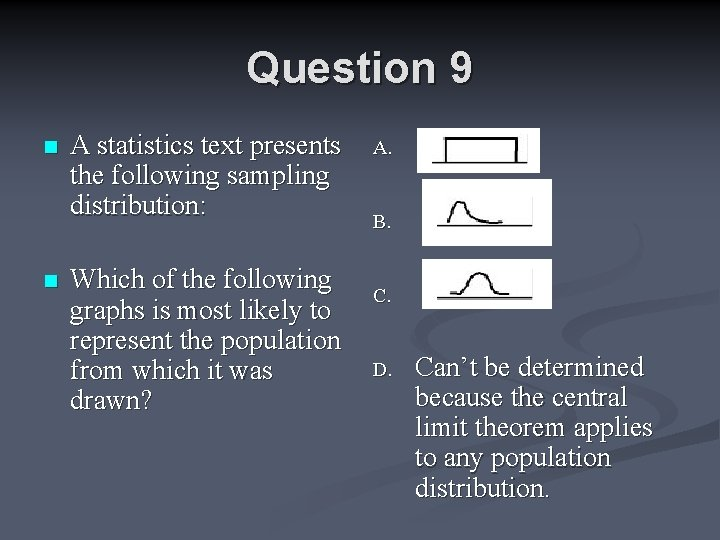Question 9 n n A statistics text presents the following sampling distribution: Which of the following graphs is most likely to represent the population from which it was drawn? A. B. C. D. Can’t be determined because the central limit theorem applies to any population distribution.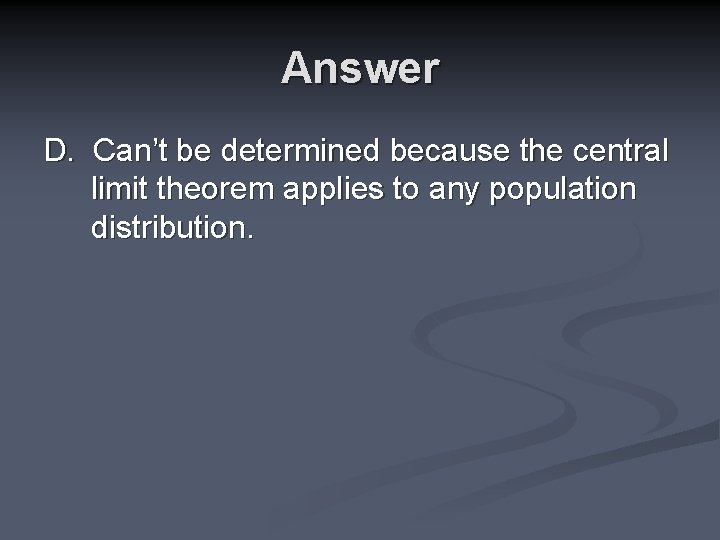Answer D. Can’t be determined because the central limit theorem applies to any population distribution.Key Ideas of it n What are the key ideas of the Central Limit Theorem?